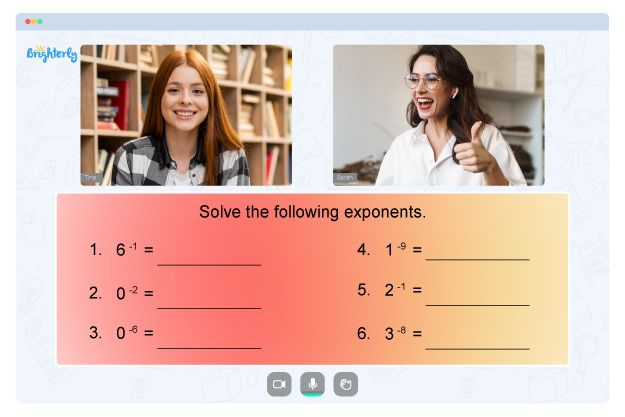# Solving Proportions Worksheets

Students must first understand the idea of proportion and then proceed step by step to solve the proportion worksheet. These worksheets keep students interested and help them solve some of the most challenging proportion problems.

A wide range of proportion problems from real-life scenarios is found in the percent proportion worksheet. The answers will be in numeric, fractional, or decimal form. You can also solve proportion worksheets that include variables in algebraic expressions.

## How to Solve a Proportion Worksheet

Solving any worksheets, like a 7th grade proportions worksheet, allows students to face real-life questions on proportion. A worksheet’s outline shows several methods for solving proportion-based problems. The worksheet exercise page begins with an example of a problem that shows that the cross-product and the inverse process are used to solve for variables of two equal ratios.

Solving proportion is an essential foundational skill for reasoning with balance in real-life situations. Each proportion problem has a denominator of equal ratio set or ratios of two and three-digit numbers. Students will then apply what they have learned to real-life problems using variables.

Math for Kids

Is Your Child Struggling With Math?
1:1 Online Math Tutoring## Benefits of Solving Proportion Worksheets

The best way to learn any topic is by practicing it. These math worksheets provide numerous questions for this purpose. In addition, a wide variety of questions are available and help strengthen a child’s knowledge of the topic.

The concept of proportions is used in several real-life problems related to profit loss and other basic calculations. Students who are well versed in solving proportions can attempt such problems. Hence, solving proportions worksheets is a perfect way for students to master the topic.### Solving Proportions Worksheets PDF

Solving Proportions Worksheet### Solving Proportions Worksheets PDF

Proportions Worksheet### Solving Proportions Worksheets PDF

Percent Proportion Worksheet### Solving Proportions Worksheets PDF

A free proportions worksheet will help you set up and solve proportions representing everyday situations involving integers and fractions. An answer key accompanies each math worksheet, is printable, and can be customized to fit a kid’s needs. The best feature of these worksheets is that they are flexible, which enables grade school students to work at comfortable paces.

### More Proportions Worksheets

Need help with Numbers?• Is your child finding it hard to master the concept of numbers?
• An online tutor could be of help.

Does your child struggle to grasp numbers? Try studying with an online tutor.